## 4.3Fundamentals of boundary conditions

The speciﬁcation of boundary conditions is one of the most challenging tasks in setting up a CFD simulation. The range of possible boundary conditions is endless, to cover all of the potential applications and physics.

Setting boundary conditions is not an exact science but is guided by an basic speciﬁcation using the ﬁxed value (Dirichlet) and ﬁxed gradient (Neumann) conditions introduced in Sec. 4.2 .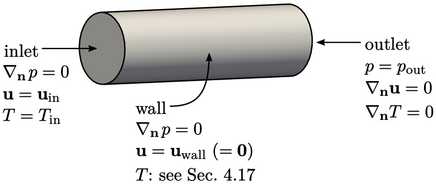The ﬁgure above shows the basic speciﬁcation for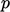,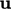and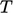for incompressible subsonic ﬂow, e.g. described by Eq. (2.47 ), Eq. (2.48 ) and Eq. (2.65 ).

The boundary conditions forandrequire particular attention since they are coupled. The conditions onare independent ofandand are representative of other transported scalar ﬁelds, e.g. turbulent kinetic energy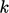.

The speciﬁcation for inlets and outlets summarises as:

• zero gradient on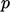at an inlet, ﬁxed value on other variables;
• ﬁxed value onat an outlet, zero gradient on other variables.

### Propagation of disturbances

A disturbance in a ﬂow is simply any change from an equilibrium or steady solution. A disturbance at one location travels, or propagates, through the ﬂuid.

The combination of boundary conditions at open boundaries, i.e. those excluding walls, relates to the propagation of disturbances. While disturbances are transported by advection with the ﬂow, they propagate as waves at the speed of sound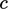.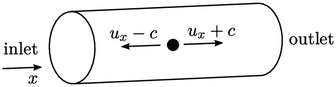Sound waves can propagate disturbances against the direction of ﬂow if it is subsonic, i.e.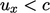. Disturbances must be able to propagate outwards through the inlet, which requires one variable not to be prescribed at the inlet. Similarly, they must be able propagate inwards from the outlet, which requires one variable to be prescribed at the outlet.

A pressure equation, combining mass and momentum conservation, describes wave propagation. For an incompressible ﬂuid the wave speed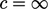, since Eq. (2.48 ) contains no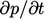term. A disturbance at any point inﬂuences the solution everywhere in the domain instantaneously, as discussed in Sec. 2.22 .

Pressure is then logically the variable on which to specify the boundary conditions to support wave propagation. Pressure is therefore: prescribed at the outlet, i.e. we specify ﬁxed value condition; not prescribed at the inlet, i.e. we specify a ﬁxed gradient condition, usually set to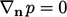.

Notes on CFD: General Principles - 4.3 Fundamentals of boundary conditions